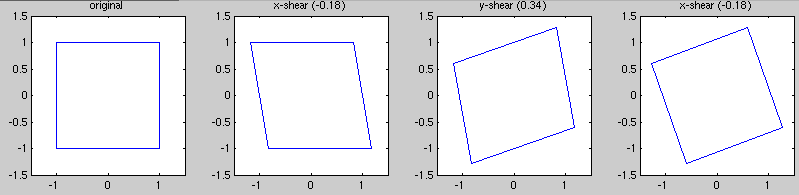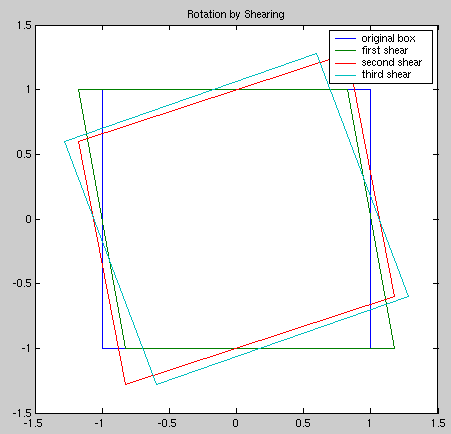# Rotation by Shearing

(Or, "how do I rotate a bitmap?")

What follows are my own notes on Alan Paeth's ``A Fast Algorithm for General Raster Rotation,'' as published in the proceedings of Graphics Interface '86. This is a very popular algorithm for image rotation, used by many libraries such as ImageMagick, pnmrotate, etc. Initially I hoped that this scheme would be suitable for use in scientific data processing where arrays must be rotated, but now I believe that this scheme is unsuitable due to the poor interpolation. I also question whether this is any faster than bilinear interpolation when run on general purpose hardware (due to the large amount of data movement); however I do not yet have any quantitative measurements. These concerns aside, the decomposition of a rotation into three shears is interesting in its own right. — Tobin 2002-07-07

A two dimensional shear operation axis has the following matrix representations (one shear matrix for a shear parallel to the X axis, and another for a shear parallel to the Y axis):

`Shear-X(α)` = [1 α ; 0 1 ]
`Shear-Y(β)` = [1 0 ; β 1 ]

Thus a shear in the X direction produces y':=y (unchanged) and x':=x+αy.

It turns out that any orthogonal transformation can be realised by a combination of a shear along one axis, then a shear along the other axis, followed by another shear along the first axis: (although that is not proved here)

`ShearX(α) ShearY(β) ShearX(γ)` = [1 α ; 0 1 ] [1 0; β 1 ] [1 γ ; 0 1 ] = [1+αβ, α+γ+αβγ ; β, 1+βγ ]

Doing a rotation by performing three shear operations might be advantageous, because it's easy to do a shear operation. To do a shear operation on a raster image (that is to say, a bitmap), we just shift all the pixels in a given row (column) by an easy-to-calculate displacement. But in order to do a rotation using shears, we'll have to be able to calculate the necessary values of α, β, and γ from the rotation angle Θ.

Recall the familiar rotation matrix:

`Rotate(Θ)` = [ cos(Θ), -sin(Θ) ; sin(&Theta), cos(&Theta) ]

Set the rotation matrix equal to the product of the three shears:

[1+αβ, α+γ+αβγ ; β, 1+βγ ] = [ cos(Θ), -sin(Θ) ; sin(&Theta), cos(&Theta) ]

Solve for α, β, & γ in terms of Θ:

&beta = sin(Θ)

1+αβ=cos(Θ)
1+αsin(Θ)=cos(Θ)
α=(cos(Θ)-1)/sin(Θ)
α=-tan(Θ/2)

1+αβ=1+βγ
γ=α

Thus we have α=γ=-tan(Θ/2) and &beta = sin(Θ).

### Example

Let Θ=20°. Then α~-0.18 and β~0.34. Here you can see what a square looks like as the three shears are applied:Just for fun we can superimpose the original box, the intermediate results, and the final image:That's it!

### Implementation

Alan Paeth presents code in a Pascal-like language to do a shear in an efficient manner, using a simple approximation to do some interpolation (see his paper for the details of the approximation). Here's the code:

```PROCEDURE xshear(shear, width, height)
FOR y:= 0 TO height-1 DO
skew    := shear * (y + 0.5);
skewi   := floor(skew);
skewf   := frac(skew);  /* = skew - skewi */
oleft   := 0;
FOR x:= 0 TO width-1 DO
pixel := P(width-x, y);
left  := pixmult(pixel, skewf);
/* pixel - left = right */
pixel := pixel - left + oleft;
P(width-x+skewi, y) := pixel;
oleft := left;
OD
P(skewi, y) := oleft;
OD
END
```

You can just replace the `pixmult` function with multiplication in most cases; its function is just to weight the given pixel value for the "`skewf`" fraction. The array to be sheared is given as the variable `P`, and `shear` is the shear factor (i.e. α, β, or γ). To make this useful you'll have to incorporate a change-of-origin to the center of rotation, and a suitable displacement to deal with negative indicies.

Here's the beginnings of an implementation in matlab: (xshear.m)

```function data_out = xshear(data,shear,antialias)
% function data = xshear(data,shear)
%
% \$Id: xshear.m,v 1.1 2002/07/06 17:38:37 tobin Exp \$

the_size = size(data);
width = the_size(1);
height = the_size(2);

for y=1:height,
skew = shear * y;            % how much to shift this line
skewi = floor(skew);         % integer part
skewf = skew - skewi;        % fractional part
oleft = 0;
for x=width:-1:1,            % from right to left...
pixel = data(x, y);        % sample the pixel
if antialias,
left = pixel * skewf;
else
left = 0;
end

pixel = (pixel - left) + oleft;
if (x+skewi > 0),
data_out(x+skewi,y) = pixel;
end
oleft = left;
end
if (skewi+1 > 0),
data_out(skewi+1,y) = oleft;
end
end
```

Tobin Fricke